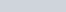# Lagrange’s equations in time-space

This post is based on the article Deriving Lagrange’s equations using elementary calculus by Josef Hanc, Edwin F. Taylow, and Slavomir Tuleja (AJP 72(4) 2004), which provides a derivation of Lagrange’s equations from the principle of least action using elementary calculus.

A tempicle moves along the t axis with potential lethargy W(t), which is location-independent. For this special case the Lagrange function or Lagrangian M has the form:

Lagrange’s Equation

Begin by fixing events 1 and 3 and varying time, t, in way similar to varying location, x, in the article.The average times and lenticities along segments A and B are

The above expressions are all functions of the single variable t. For later use take the derivatives with respect to t:

Let MA and MB be the values of the Lagrangian on segments A and B, respectively, and label the summed action across these two segments as TAB:

QAB = MA Δx + MB Δx.

The principle of least action requires that the coordinates of the middle event t be chosen to  yield the smallest value of the action between the fixed events 1 and 3. If we sest the derivative of TAB with respect to t equal to zero and use the chain rule, we obtain

Next substitute the derivatives above into this equation, divide through by Δx, and regroup the terms to obtain

To first order, the first term above is the average value of ∂M/∂t on the two segments A and B. In the limit Δx→0, this term approaches the value of the partial derivative at t. In the same limit, the second term above becomes the location derivative of the partial derivative of the Lagrangian with respect to lenticity d(∂M/∂w)/dt. Therefore in the limit Δx→0, the above equation becomes the Lagrange equation in t:

The additive property of the action implies that the above equation is valid for every adjacent pair of segments.

Levamentum

Consider the case in which the Lagrangian does not depend explicitly on the t coordinate of the tempicle. Because it does not appear in the Lagrangian, the t coordinate is “ignorable” of “cyclic.” For a Lagrangian that is only a function of the lenticity, M = M(w), Lagrange’s equation above tells us that the location derivative of ∂M/∂w is zero. From the first equation above, we find that ∂M/∂w = nw, which implies that the levamentum, q = vw, is a constant of the motion.

If we repeat the derivation in the previous section with M = M(w), we obtain from the plinciple of least action

Substitute the derivatives and rearrange the terms to find:

orAgain we can use the arbitrary chronation of segments A and B along the world timeline to conclude that the levamentum q is always a constant of the motion on the world timeline.

Lethargy

Consider a tempicle in a location-independent potential W(t). Now vary the location of the middle event, rather than its chronation, requiring that this location be chosen to minimize the action.For simplicity, choose the t increments to be equal, with the value Δt. Keep the spatial coordinates of all three events fixed while varying the location coordinate of the middle event and obtain

These expressions are functions of the single variable x, with respect to which we take the derivatives

and

Despite the form of the previous equations, the derivatives of lenticities are not relentations, because the t separations are held constant while the location is varied.

As before,

We find the value of the location x for the action to be a minimum by setting the derivative of QAB equal to zero:

If we substitute the derivatives into this equation and rearrange the result, we find

Because the action is additive this equation is valid for every segment of the world timeline and identifies the function wM/∂wM as a constant of the motion. By substituting the first equation for the Lagrangian into wM/∂wM and carrying out the partial derivatives, we can show that the constant of the motion corresponds to the total lethargy D = K + W.